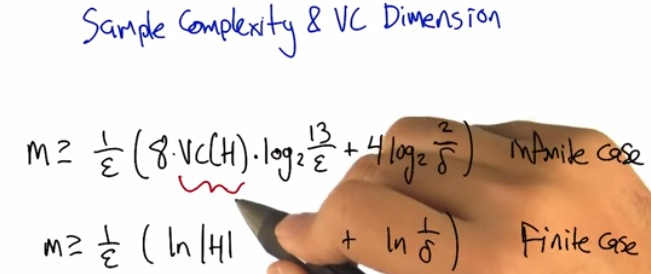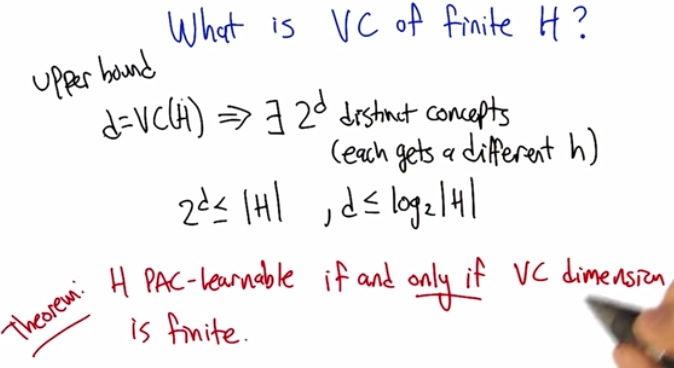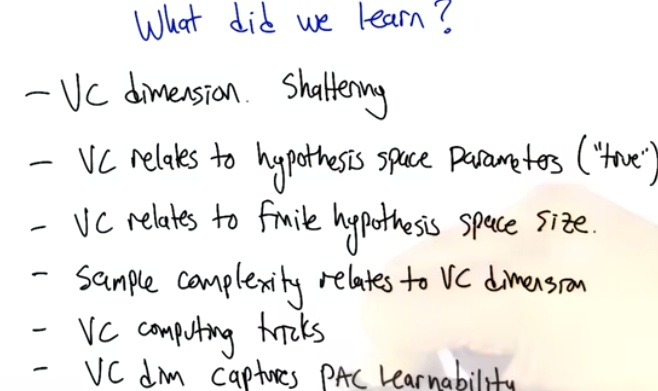How to count m if the set of hypothesis is infinity?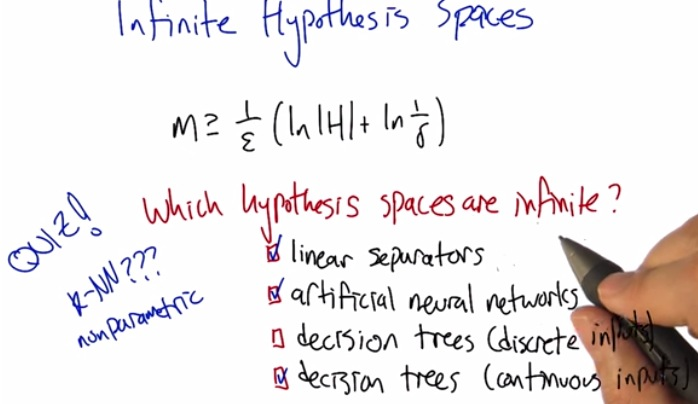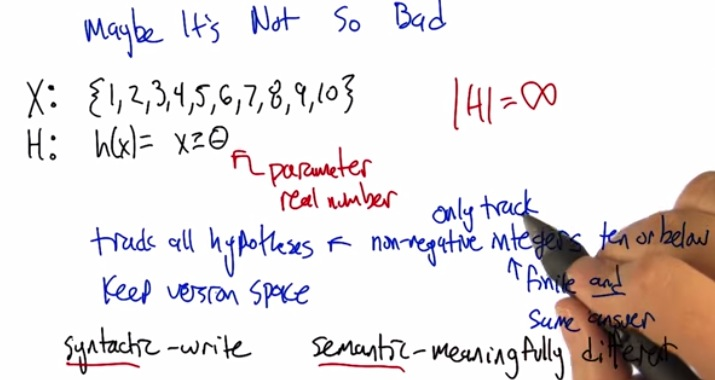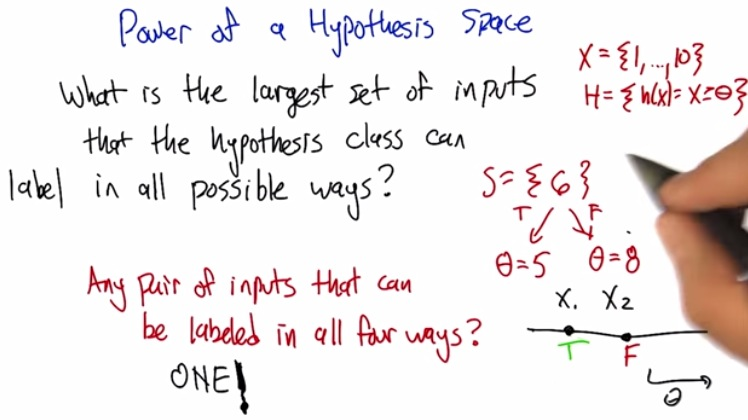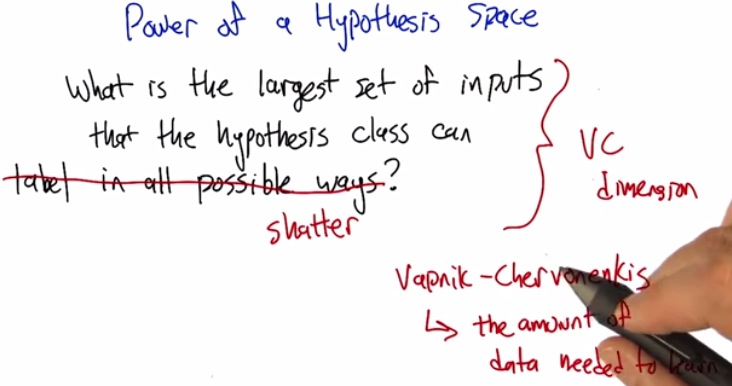• VC stated that eventhough the hypothesis space is infinite, as long as the sample is finite, then we can know how much the samples do we need.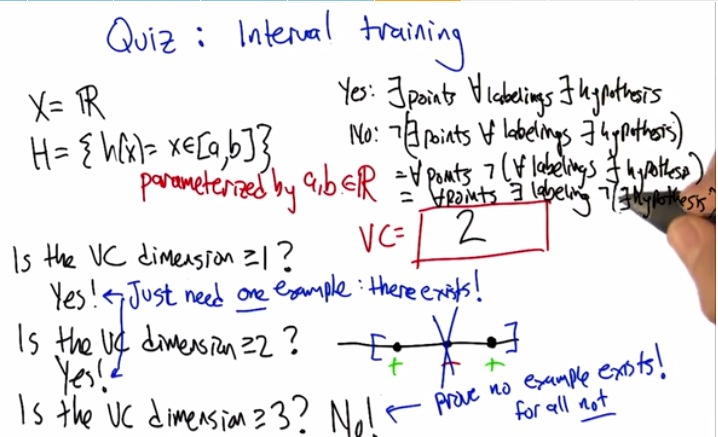• Proving lower bound is easier than proving an upper bound. Means that in this case we only need to to prove at least one example, than using max example
• For VC dimension, we need one example, but to prove that, test infinite number of hypothesis.
• In the vc dimension = 2, we know that we still get positive, in setting the limit [], between those two
• But in the case vc dimension = 3, one example exists that we can't use the VC, so therefore at least 2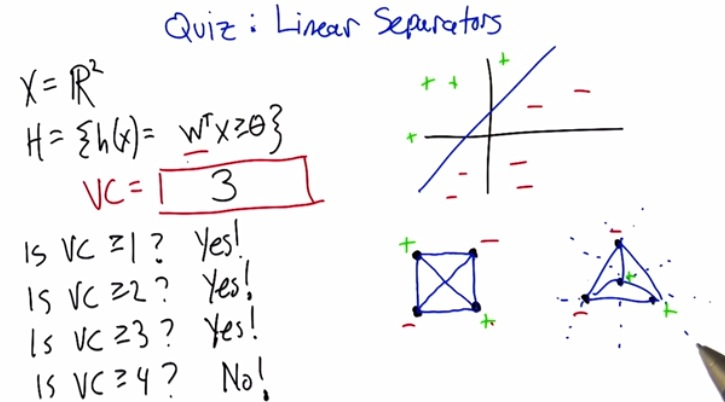• Here we have R2 dimension, and as an input, weight parameters wT*X and the output will be labeled by some hypothesis theta
• R2 will get 3 VC Dimensions.
• while R1 get 2VC, R2 get 3VC, does this get directly correlated by nVC = Rn-1?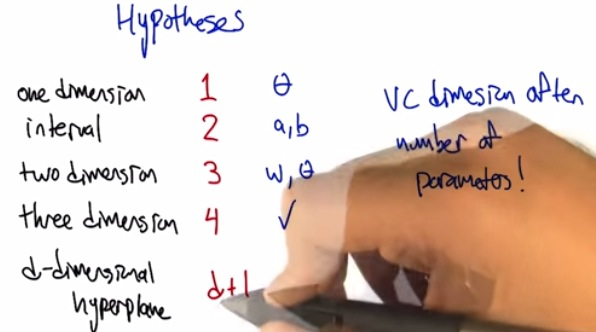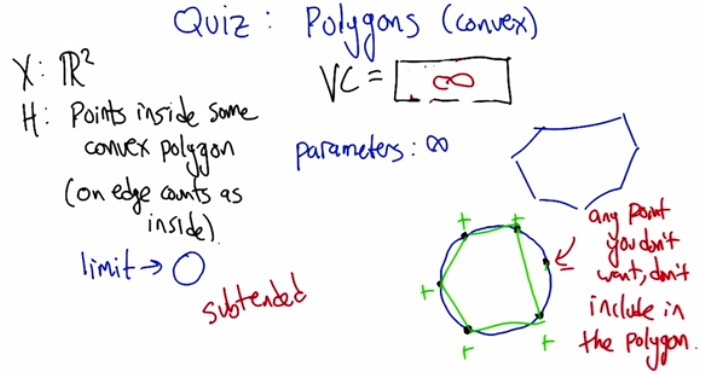• VC dimension directly correlated with the numbers of parameters.